How to Learn in 24 Hours?

 Need Help? M-F: 9am-5pm(PST): Toll-Free: (877) RAPID-10 or 1-877-727-4310 24/7 Online Technical Support: The Rapid Support Center vip@rapidlearningcenter.com Secure Online Order:Need Proof? Testimonials by Our Users

 Rapid Learning Courses: MCAT in 24 Hours (2021-22) USMLE in 24 Hours (Boards) Chemistry in 24 Hours Biology in 24 Hours Physics in 24 Hours Mathematics in 24 Hours Psychology in 24 Hours SAT in 24 Hours ACT in 24 Hours AP in 24 Hours CLEP in 24 Hours DAT in 24 Hours (Dental) OAT in 24 Hours (Optometry) PCAT in 24 Hours (Pharmacy) Nursing Entrance Exams Certification in 24 Hours eBook - Survival Kits Audiobooks (MP3)

 Tell-A-Friend: Have friends taking science and math courses too? Tell them about our rapid learning system.Home »  Mathematics »  Intermediate Algebra

Sequences and Series

 Topic Review on "Title": The definition of infinite sequences:  An (infinite) sequence of real numbers is a function from the positive integers n into real numbers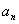,Limit of a sequence: A sequence of real numbers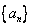converges to the numberif, for any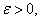there is a positive integer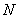such thatfor any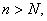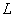is called the limit of the sequence. Convergence of Cauchy sequences: A sequence of real numbers converges if and only if it is a Cauchy sequence. Subsequences of a sequence: Subsequences are formed when we have a strictly increasing sequence of positive integers. Convergence tests: The comparison test, ratio test, root test, integral test and absolute/ conditional convergence test are the tests that are used to determine the convergence of series.

Rapid Study Kit for "Title":
 Flash Movie Flash Game Flash Card Core Concept Tutorial Problem Solving Drill Review Cheat Sheet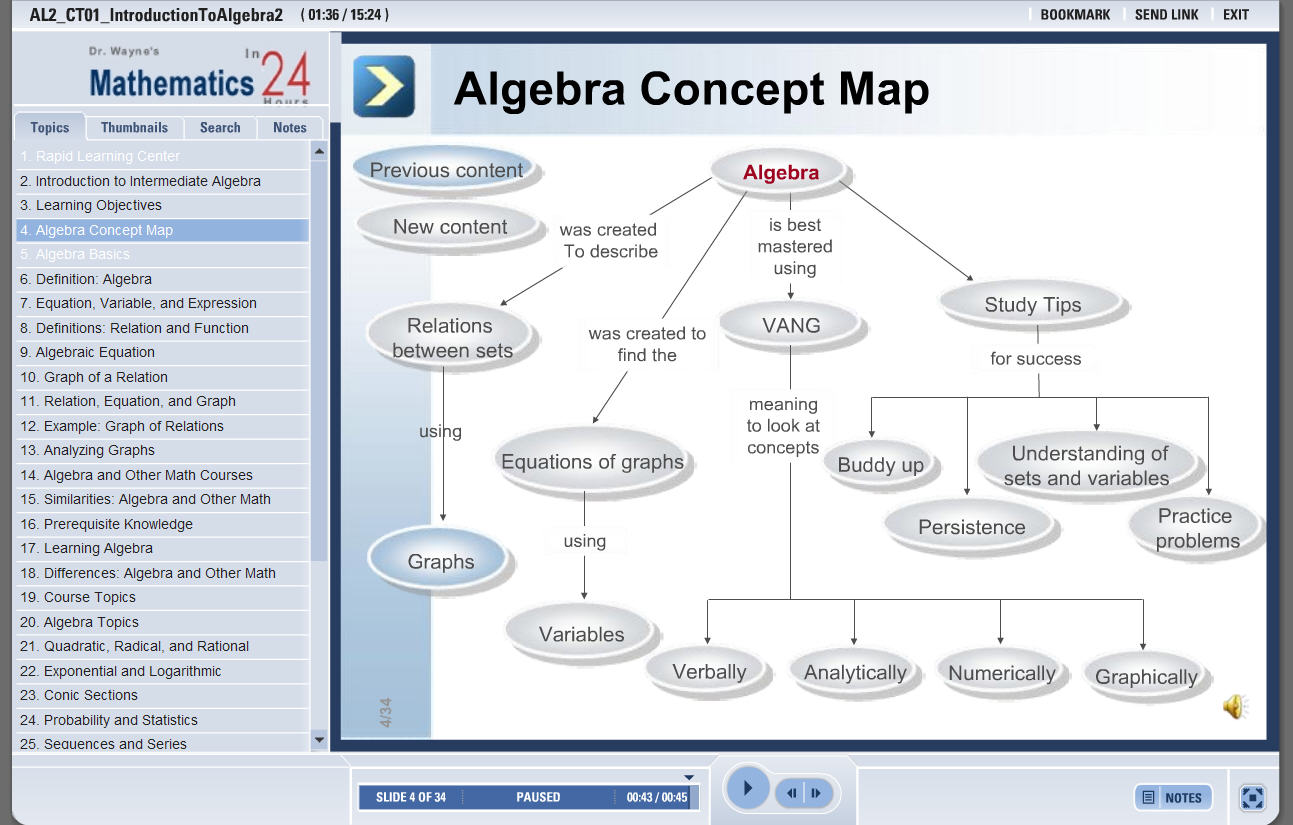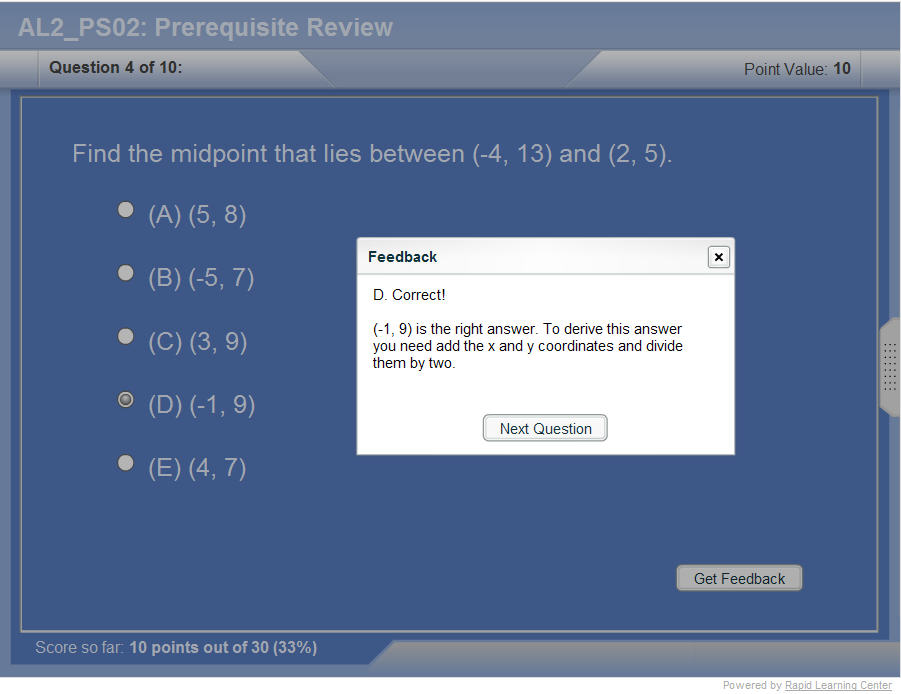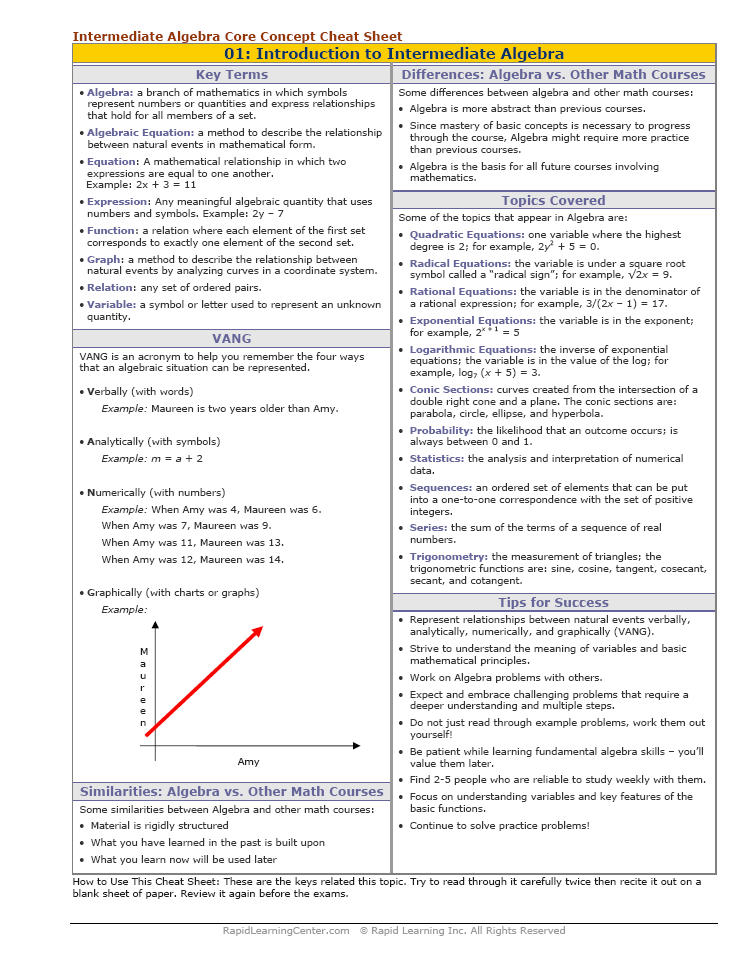"Title" Tutorial Summary : Infinite sequences and series are mentioned in this tutorial. The basic operations and properties of infinite sequences are presented in some of the examples. The limit of sequences can be found using some type of theorem. Limits can be approximated using some of the properties of sequences. The uniqueness and convergence of sequences need to be discussed before the concept of subsequences is defined. Special sequences such as Cauchy sequences are mentioned in this tutorial to reinforce the efficiency of sequences and their properties.

 Tutorial Features: Specific Tutorial Features: • The convergence of sequences is shown using graphs. • Step by step analysis of how a series can be found to be convergent using the convergence series tests. Series Features: • Concept map showing inter-connections of new concepts in this tutorial and those previously introduced. • Definition slides introduce terms as they are needed. • Visual representation of concepts • Animated examples—worked out step by step • A concise summary is given at the conclusion of the tutorial.

 "Title" Topic List: Sequences that are infinite Arithmetic and geometric progression Sequences and their definition Recursive relations Limit of a sequence Divergence of sequences Operations of a limit Bounded and monotonic sequences Cauchy sequences and their definition Convergence of Cauchy sequences Subsequences Infinite series Arithmetic Sum Geometric Series Series Properties Comparison test Ratio, root, integral, p-series and alternating series test

See all 24 lessons in Intermediate Algebra, including concept tutorials, problem drills and cheat sheets:
Teach Yourself Intermediate Algebra Visually in 24 Hours